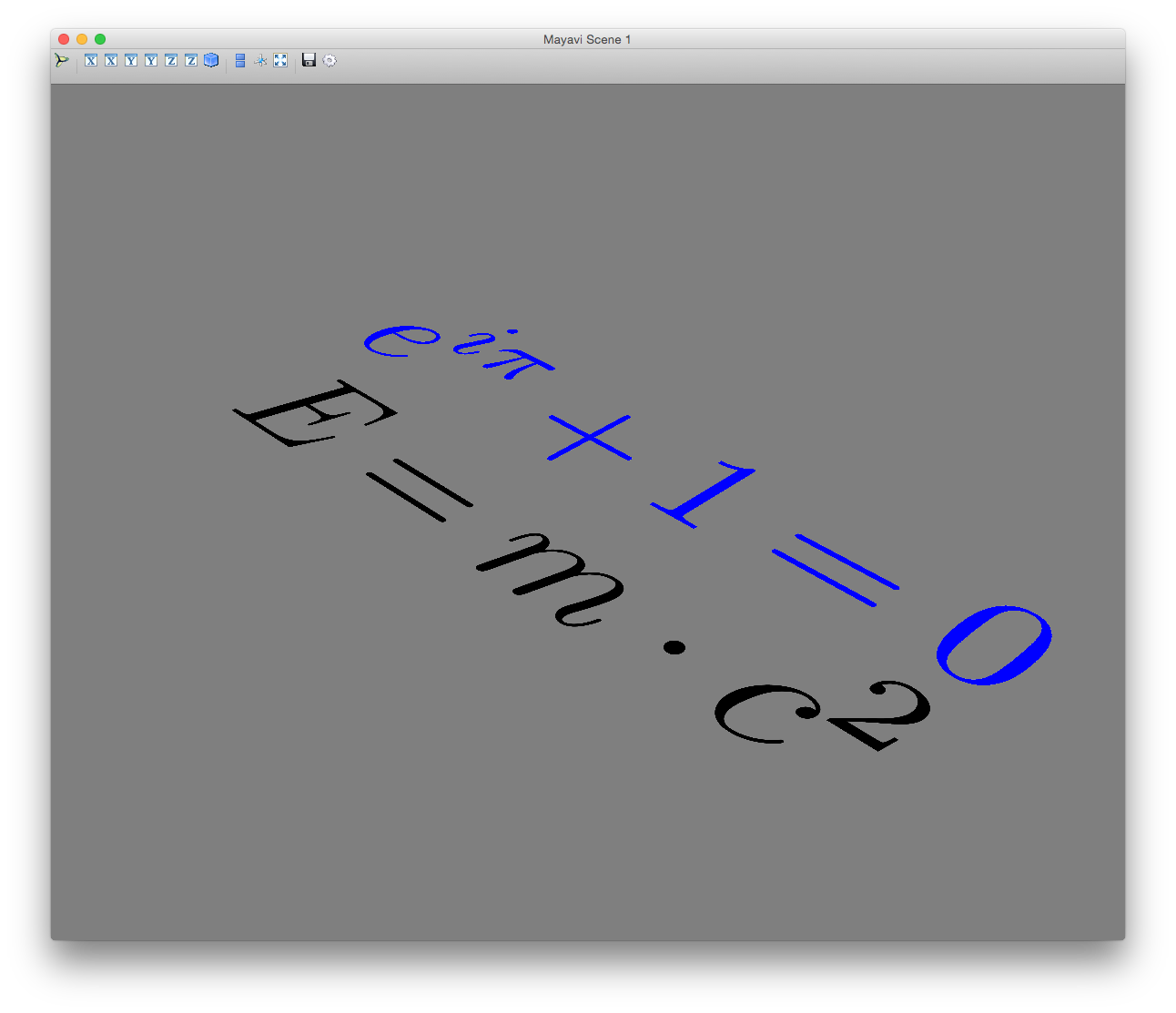# Using LaTeX formulas in Mayavi (Python)

Mayavi does not support using LaTeX directly. However, it is possible to render LaTeX output to a png image file with external tools and then load this image into Mayavi as a colormap. To simplify the conversion steps, we offer a python module (mlab_latex_out.py) that takes a LaTeX math string and adds the rendered formulato the current Mayavi scene.

Here is an example how to use the module:

from mayavi import mlab
from mlab_latex_out import mlab_imshow_latex

latex_obj = mlab_imshow_latex(r'E = m \cdot c^2')
latex_obj.actor.scale = [0.75, 0.75, 0.75]
latex_obj.actor.position = [250, 0, 0]
latex_obj = mlab_imshow_latex(r'e^{i\pi} + 1 = 0', textcolor=(0, 0, 255)) # textcolor is a rgb tuple
latex_obj.actor.scale = [0.75, 0.75, 0.75]
latex_obj.actor.position = [-250, 0, 0.01]
mlab.show()